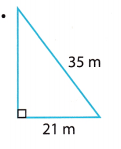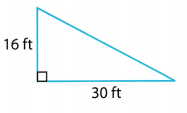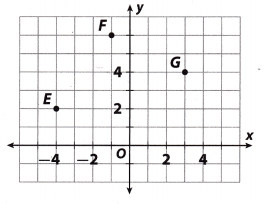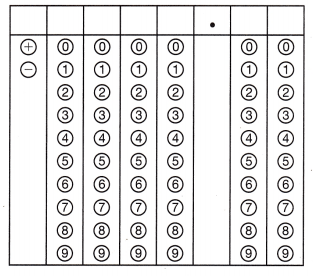Refer to our Texas Go Math Grade 8 Answer Key Pdf to score good marks in the exams. Test yourself by practicing the problems from Texas Go Math Grade 8 Module 8 Quiz Answer Key.

8.1 The Pythagorean Theorem

Find the length of the missing side.

Question 1.Let b = 21 and c = 35 Using the Pythagorean Theorem, we have:
a2 + b2 = c2
a2 + 212 = 352
a2 + 441 = 1225
a2 + 441 – 441 = 1225 – 441
a2 = 784
a = $$\sqrt {281}$$
The length of the missing side is 28 m.

Question 2.Let a = 16 and b = 30 Using the Pythagorean Theorem, we have:
a2 + b2 = c2
162 + 302 = c2
256 + 900 = c2
1156 = c2
c = $$\sqrt {1156}$$
c = 34
The length of the missing side is 34 m.

8.2 Converse of the Pythagorean Theorem

Tell whether each triangle with the given side lengths Is a right triangle.

Question 3.
11, 60, 61 _____________
Let a = 11, b = 60 and c = 61. Using the converse of the Pythagorean Theorem, we have:
a2 + b2 = c2
112 + 602 = 612
121 + 3600 = 3721
3721 = 3721
True
Since 112 + 602 = 612, the triangle is a right triangle.

Question 4.
9, 37, 40 _____________
Let a = 9, b 37 and c = 40. Using the converse of the Pythagorean Theorem, we have:
a2 + b2 = c2
92 + 372 = 402
81 + 1369 = 1600
1450 = 1600
False
Since 92 + 372 ≠ 402, the triangle is not a right triangle.

Question 5.
15, 35, 38 _____________
Let a = 15,b = 35, and c = 38.
a2 + b2 = c2 …………… (1)
152 + 352 = 382 (Substitute into the formula) ……………. (2)
225 + 1225 = 1444 (Simplify) …………. (3)
1450 ≠ 1444 (Add) …………. (4)
Since 152 + 352 ≠ 382, the triangle is not a right triangle by the converse of the Pythagorean Theorem.

Question 6.
28, 45, 53 _____________
Let a = 28, b = 45 and c = 53. Using the converse of the Pythagorean Theorem, we have:
a2 + b2 = c2
282 + 452 = 532
784 + 2025 = 2809
2809 = 2809
True
Since 282 + 452 = 532 the triangle is a right triangle.

Question 7.
Keelie has a triangular-shaped card. The lengths of its sides are 4.5 cm, 6 cm, and 7.5 cm. Is the card a right triangle?
Let a = 4.5, b = 6 and c = 7.5. Using the converse of the Pythagorean Theorem, we have:
a2 + b2 = c2
4.52 + 62 = 7.52
20.25 + 36 = 56.25
56.25 = 56.25
True
Since 4.52 + 62 = 7.52 , the card is a right triangle.

8.3 Distance Between Two Points

Find the distance between the given points. Round to the nearest tenth.Question 8.
A and B _____________
Using the Distance Formula, the distance d between the points A(-2, 3) and B(4, 6) is:
d = $$\sqrt{\left(x_{2}-x_{1}\right)^{2}+\left(y_{2}-y_{1}\right)^{2}}$$
d = $$\sqrt{(4-(-2))^{2}+(6-3)^{2}}$$
d = $$\sqrt{(6)^{2}+(3)^{2}}$$
d = $$\sqrt{36+9}$$
d = $$\sqrt{45}$$
Rounding the answer to the nearest tenth:
d ≈ 6.7
The distance between points A and B is approximately 6.7 units.

Question 9.
B and C. _____________
Using the Distance Formula, the distance d between the points B(4, 6) and C(3, -1) is:
d = $$\sqrt{\left(x_{2}-x_{1}\right)^{2}+\left(y_{2}-y_{1}\right)^{2}}$$
d = $$\sqrt{(3-4)^{2}+(-1-6)^{2}}$$
d = $$\sqrt{(-1)^{2}+(-7)^{2}}$$
d = $$\sqrt{1+49}$$
d = $$\sqrt{50}$$
Rounding the answer to the nearest tenth:
d ≈ 7.1
The distance between points B and C is approximately 7.1 units.

Question 10.
A and C _____________
Using the Distance Formula, the distance d between the points A(-2, 3) and C(3, -1) is:
d = $$\sqrt{\left(x_{2}-x_{1}\right)^{2}+\left(y_{2}-y_{1}\right)^{2}}$$
d = $$\sqrt{(3-(-2))^{2}+(-1-3)^{2}}$$
d = $$\sqrt{(5)^{2}+(-4)^{2}}$$
d = $$\sqrt{25+16}$$
d = $$\sqrt{41}$$
Rounding the answer to the nearest tenth:
d ≈ 6.4
The distance between points A and C is approximately 6.4 units.

Essential Question

Question 11.
How can you use the Pythagorean Theorem to solve real-world problems?
We can use the Pythagorean Theorem to find the length of a side of a right triangle when we know the lengths of the other two sides. This application is usually used in architecture or other physical construction projects. For example, it can be used to find the length of a ladder, if we know the height of the wall and the distance on the ground from the wall of the ladder.

Texas Go Math Grade 8 Module 8 Mixed Review Texas Test Prep Answer Key

Selected Response

Question 1.
What is the missing length of the side?(A) 9 ft
(B) 30 ft
(C) 39 ft
(D) 120 ft
By using the Pythagorean theorem,
a² + b² = c²
80² + b² = 89²
b² = 89² – 80²
b = √89² – 80²
b = √9²
b = 9 ft
Option A is the correct answer.

Question 2.
Which relation does not represent a function?
(A) (0, 8), (3, 8), (1, 6)
(B) (4, 2), (6, 1), (8, 9)
(C) (1, 20), (2, 23), (9, 26)
(D) (0, 3), (2, 3), (2, 0)
Answer: D is the relation that does not represent a function.

Question 3.
Two sides of a right triangle have lengths of 72 cm and 97 cm. The third side is not the hypotenuse. How long is the third side?
(A) 25 cm
(B) 45 cm
(C) 65 cm
(D) 121 cm
(C) 65 cm

Explanation:
Let b = 72 and c = 97, Using the Pythagorean Theorem, we have:
a2 + b2 = c2
a2 + 722 = 972
a2 + 5184 = 9409
a2 + 5184 – 5184 = 9409 – 5184
a2 = 4225
a = 65

Question 4.
What is the distance between point F and point G?(A) 4.5 units
(B) 5 units
(C) 7.3 units
(D) 20 units
(A) 4.5 units

Explanation:
Write the coordinates of the points F(-1, 6) and G(3, 4), and find the distance between F and G. Using the Distance FormulaQuestion 5.
A flagpole is 53 feet tall. A rope is tied to the top of the flagpole and secured to the ground 28 feet from the base of the flagpole. What is the length of the rope?
(A) 25 feet
(B) 45 feet
(C) 53 feet
(D) 60 feet
(D) 60 feet

Explanation:
A flagpole, rope and the length of secure point from the flagpole are forming the right triangle, where the rope is a hypotenuse Let a = 53 ft and b = 28 ft Use Pythagorean Formula to find length of the rope c.
a2 + b2 = c2 ……………… (1)
c2 = 532 + 282 (Substitute into formula) …………….. (2)
c2 = 2809 + 784 (Simplify) …………… (3)
c2 = 3593 (Add) …………. (4)
c = 59.94163 (Take the square root from both sides) …………. (5)
c ≈ 60 ft (Round to the nearest whole number) …………. (6)

Question 6.
Which set of lengths are not the side lengths of a right triangle?
(A) 36, 77, 85
(B) 20, 99, 101
(C) 27, 120, 123
(D) 24, 33, 42
(D) 24, 33, 42

Explanation:
Check if side lengths in option A form a right triangle.
Let a = 36, b = 77 and c = 85. Using the converse of the Pythagorean Theorem, we have:
a2 + b2 = c2
362 + 772 = 852
1296 + 5929 = 7225
7225 = 7225
True
Since 362 + 772 = 852, the triangle is a right triangle.

Check if side lengths in option B form a right triangle.
Let a = 20, b = 99 and c = 101. Using the converse of the Pythagorean Theorem, we have:
a2 + b2 = c2
202 + 992 = 1012
400 + 9801 = 10201
10201 = 10201
True
Since 202 + 992 = 1012, the triangle is a right triangle

Check if side Lengths in option C form a right triangle
Let a = 27, b = 120 and c = 123. Using the converse of the Pythagorean Theorem, we have:
a2 + b2 = c2
272 + 1202 = 1232
729 + 14400 = 15129
15129 = 15129
True
Since 272 + 1202 = 1232, the triangle is a right triangle.

Check if side lengths in option D form a right triangle
Let a = 24, b = 33 and c = 42. Using the converse of the Pythagorean Theorem, we have:
a2 + b2 = c2
242 + 332 = 422
576 + 1089 = 1764
1665 = 1764
False
Since 242 + 332 ≠ 422, the triangle is not a right triangle.

Question 7.
Which is an irrational number?
(A) 5.4
(B) $$\sqrt {7}$$
(C) -13
(D) $$\frac{2}{3}$$
Answer: (D) $$\frac{2}{3}$$
Explanation:
$$\frac{2}{3}$$ = 0.66666…
0.66666… is a recurring number.
So, Option D is the correct answer.

Question 8.
A triangle has one right angle. What could the measures of the other two angles be?
(A) 25° and 65°
(B) 30° and 15°
(C) 55° and 125°
(D) 90° and 100°
A triangle has one right angle
Sum of three angles = 180°
25° + 65° + 90° = 180
So, option A is the correct answer.

Gridded Response

Question 9.
A right triangle has legs that measure 1.5 centimeters and 2 centimeters. What is the length of the hypotenuse in centimeters?